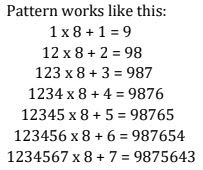# NCERT Solutions for Chapter 2 Whole Numbers Class 6 Maths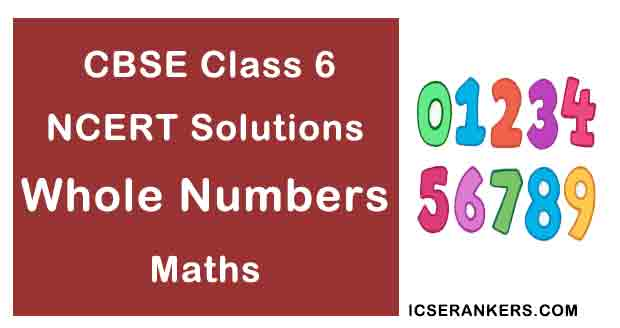### Whole Numbers Questions and Answers

 Chapter Name Whole Numbers NCERT Solutions Class CBSE Class 6 Textbook Name Maths Related Readings NCERT Solutions for Class 6NCERT Solutions for Class 6 Maths Summary of Whole Numbers

Exercise 2.1

Question 1:  Write the next three natural numbers after 10999.

Solution

10,999 + 1 = 11,000

11,000 + 1 = 11,001

11,001 + 1 = 11,002

Question 2: Write the three whole numbers occurring just before 10001.

Solution

10,001 – 1 = 10,000

10,000 – 1 = 9,999

9,999 – 1 = 9,998

Question 3: Which is the smallest whole number?

Solution

‘0’ (zero) is the smallest whole number.

Question 4: How many whole numbers are there between 32 and 53?

Solution

53 – 32 – 1 = 20

There are 20 whole numbers between 32 and 53.

Question 5: Write the successor of:

(a) 2440701

(b) 100199

(c) 1099999

(d) 2345670

Solution

(a) Successor of 2440701 is 2440701 + 1 = 2440702

(b) Successor of 100199 is 100199 + 1 = 100200

(c) Successor of 1099999 is 1099999 + 1 = 1100000

(d) Successor of 2345670 is 2345670 + 1 = 2345671

Question 6: Write the predecessor of:

(a) 94

(b) 10000

(c) 208090

(d) 7654321

Solution

(a) The predecessor of 94 is 94 – 1 = 93

(b) The predecessor of 10000 is 10000 – 1 = 9999

(c) The predecessor of 208090 is 208090 – 1 = 208089

(d) The predecessor of 7654321 is 7654321 – 1 = 7654320

Question 7: In each of the following pairs of numbers, state which whole number is on the left of the

other number on the number line? Also write them with the appropriate sign (>, <)

between them.

(a) 530, 503

(b) 370, 307

(c) 98765, 56789

(d) 9830415, 10023001

Solution

(a) 530 > 503;

So 503 appear on left side of 530 on number line.

(b) 370 > 307;

So 307 appear on left side of 370 on number line.

(c) 98765 > 56789;

So 56789 appear on left side of 98765 on number line.

(d) 9830415 < 10023001;

So 9830415 appear on left side of 10023001 on number line.

Question 8: Which of the following statements are true (T) and which are false (F):

(a) Zero is the smallest natural number.

(b) 400 is the predecessor of 399.

(c) Zero is the smallest whole number.

(d) 600 is the successor of 599.

(e) All natural numbers are whole numbers.

(f) All whole numbers are natural numbers.

(g) The predecessor of a two digit number is never a single digit number.

(h) 1 is the smallest whole number.

(i) The natural number 1 has no predecessor.

(j) The whole number 1 has no predecessor.

(k) The whole number 13 lies between 11 and 12.

(l) The whole number 0 has no predecessor.

(m) The successor of a two digit number is always a two digit number.

Solution

(a) False

(b) False

(c) True

(d) True

(e) True

(f) False

(g) False

(h) False

(i) True

(j) False

(k) False

(l) True

(m) False

Exercise 2.2

Question 1: Find the sum by suitable rearrangement:

(a) 837 + 208 + 363

(b) 1962 + 453 + 1538 + 647

Solution

(a) 837 + 208 + 363

= (837 + 363) + 208

= 1200 + 208

= 1408

(b) 1962 + 453 + 1538 + 647

= (1962 + 1538) + (453 + 647)

= 3500 + 1100

= 4600

Question 2: Find the product by suitable arrangement:

(a) 2 × 1768 × 50

(b) 4 × 166 × 25

(c) 8 × 291 × 125

(d) 625 × 279 × 16

(e) 285 × 5 × 60

(f) 125 × 40 × 8 × 25

Solution

(a) 2 × 1768 × 50

= (2 × 50) × 1768

= 100 × 1768

= 176800

(b) 4 × 166 × 25

= (4 × 25) × 166

= 100 × 166

= 16600

(c) 8 × 291 × 125

= (8 × 125) × 291

= 1000 × 291

= 291000

(d) 625 × 279 × 16

= (625 × 16) × 279

= 10000 × 279

= 2790000

(e) 285 × 5 × 60

= 284 × (5 × 60)

= 284 × 300

= 85500

(f) 125 × 40 × 8 × 25

= (125 × 8) × (40 × 25)

= 1000 × 1000

= 1000000

Question 3: Find the value of the following:

(a) 297 × 17 + 297 × 3

(b) 54279 × 92 + 8 × 54279

(c) 81265 × 169 – 81265 × 69

(d) 3845 × 5 × 782 + 769 × 25 × 218

Solution

(a) 297 × 17 + 297 × 3

= 297 × (17 + 3)

= 297 × 20

= 5940

(b) 54279 × 92 + 8 × 542379

= 54279 × (92 + 8)

= 54279 × 100

= 5427900

(c) 81265 × 169 – 81265 × 69

= 81265 × (169 – 69)

= 81265 × 100

= 8126500

(d) 3845 × 5 × 782 + 769 × 25 × 218

= 3845 × 5 × 782 + 769 × 5 x 5 × 218

= 3845 × 5 × 782 + 3845 × 5 × 218

= 3845 × 5 × (782 + 218)

= 3845 × 5 × 1000

= 19225000

Question 4: Find the product using suitable properties:

(a) 738 × 103

(b) 854 × 102

(c) 258 × 1008

(d) 1005 × 168

Solution

(a) 738 × 103

= 738 × (100 + 3)

= 738 × 100 + 738 × 3

= 73800 + 2214

= 76014

(b) 854 × 102

= 854 × (100 + 2)

= 854 × 100 + 854 × 2

= 85400 + 1708

= 87108

(c) 258 × 1008

= 258 × (1000 + 8)

= 258 × 1000 + 258 × 8

= 258000 + 2064

= 260064

(d) 1005 × 168

= (1000 + 5) × 168

= 1000 × 168 + 5 × 168

= 168000 + 840

= 168840

Question 5: A taxi-driver, filled his car petrol tank with 40 litres of petrol on Monday. The next day, he filled the tank with 50 litres of petrol. If the petrol costs ₹ 44 per litre, how much did he spend in all on petrol?

Solution

Petrol filled on Monday = 40 litres

Petrol filled on next day = 50 litres

Total petrol filled = 90 litres

Now,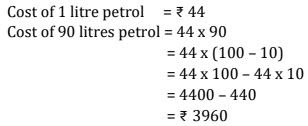Therefore, he spent ₹ 3960 on petrol.

Question 6: A vendor supplies 32 litres of milk to a hotel in a morning and 68 litres of milk in the evening. If the milk costs ₹15 per litre, how much money is due to the vendor per day?

Solution

Supply of milk in morning = 32 litres

Supply of milk in evening = 68 litres

Total supply = 32 + 68 = 100 litres

Now

Cost of 1 litre milk = ₹15

Cost of 100 litres milk = 15 × 100 = ₹1500

Therefore, ₹1500 is due to the vendor per day.

Question 7: Match the following:

 Column I Column II (i) 425 × 136 = 425 × (6 + 30 + 100) (a)Commutativity under multiplication (ii) 2 × 49 × 50 = 2 × 50 × 49 (b) Commutativity under addition (iii) 80 + 2005 + 20 = 80 + 20 + 2005 (c) Distributivity multiplication under addition

Solution

 Column I Column II (i) 425 × 136 = 425 × (6 + 30 + 100) (c) Distributivity multiplication under addition (ii) 2 × 49 × 50 = 2 × 50 × 49 (a)Commutativity under multiplication (iii) 80 + 2005 + 20 = 80 + 20 + 2005 (b) Commutativity under addition

Exercise 2.3

Question 1: Which of the following will not represent zero:

(a) 1 + 0

(b) 0 × 0

(c) 0/2

(d) 10 -10/2

Solution

(a) [1 + 0 is equal to 1]

Question 2: If the product of two whole numbers is zero, can we say that one or both of them will be zero? Justify through examples.

Solution

Yes, if we multiply any number with zero the resultant product will be zero.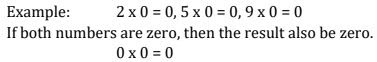Question 3: If the product of two whole number is 1, can we say that one or both of them will be 1? Justify through examples.

Solution

If only one number be 1 then the product cannot be 1.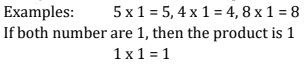Question 4: Find using distributive property:

(a) 728 × 101

(b) 5437 × 1001

(c) 824 × 25

(d) 4275 × 125

(e) 504 × 35

Solution

(a) 728 × 101

= 728 × (100 + 1)

= 728 × 100 + 728 × 1

= 72800 + 728

= 73528

(b) 5437 × 1001

= 5437 × (1000 + 1)

= 5437 × 1000 + 5437 × 1

= 5437000 + 5437

= 5442437

(c) 824 × 25

= 824 × (20 + 5)

= 824 × 20 + 824 × 5

= 16480 + 4120

= 20600

(d) 4275 × 125

= 4275 × (100 + 20 + 5)

= 4275 × 100 + 4275 × 20 + 4275 ×5

= 427500 + 85500 + 21375

= 534375

(e) 504 × 35

= (500 + 4) × 35

= 500 × 35 + 4 × 35

= 17500 + 140

= 17640

Question 5: Study the pattern:

× 8 + 1 = 9

12 × 8 + 2 = 98

123 × 8 + 3 = 987

1234 × 8 + 4 = 9876

12345 × 8 + 5 = 98765

Write the next two steps. Can you say how the pattern works?

Solution

123456 × 8 + 6 = 987654

1234567 × 8 + 7 = 9876543